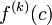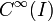Infinitely differentiable function

(diff) ← Older revision | Latest revision (diff) | Newer revision → (diff)

Definition

Definition at a point

Suppose$f$ is a function defined around a point$c \in \R$. We say that$f$ is infinitely differentiable at$c$ if the following equivalent conditions hold:

• All the higher derivatives$f^{(k)}(c)$ exist as finite numbers for all nonnegative integers$k$.
• For every nonnegative integer$k$, there is an open interval containing$c$ (possibly dependent on$k$) such that$f^{(k)}$ exists at all points on that open interval containing$c$.
• For every nonnegative integer$k$, there is an open interval containing$c$ (possibly dependent on$k$) such that$f^{(k)}$ exists and is continuous at all points on that open interval containing$c$.

Definition on an open interval or union of open intervals

Suppose$f$ is a function defined on an interval$I$ that is open but possibly infinite in one or both directions (i.e., an interval of the form$(a,b),(a,\infty),(-\infty,b),(-\infty,\infty)$). We say that$f$ is infinitely differentiable on$I$ if$f$ is infinitely differentiable at every point of$I$.

Instead of a single open interval, we could consider a union of open intervals, and use the same definition: a function is said to be infinitely differentiable on the union of open intervals if it is infinitely differentiable at every point in that union of open intervals.

The set of infinitely differentiable functions on an interval$I$ is denoted$C^\infty(I)$.

Definition without point or interval specification

If no point or interval is specified, then saying that$f$ is infinitely differentiable means that$f$ is infinitely differentiable on its entire domain. This makes sense if the domain is a union of open intervals.